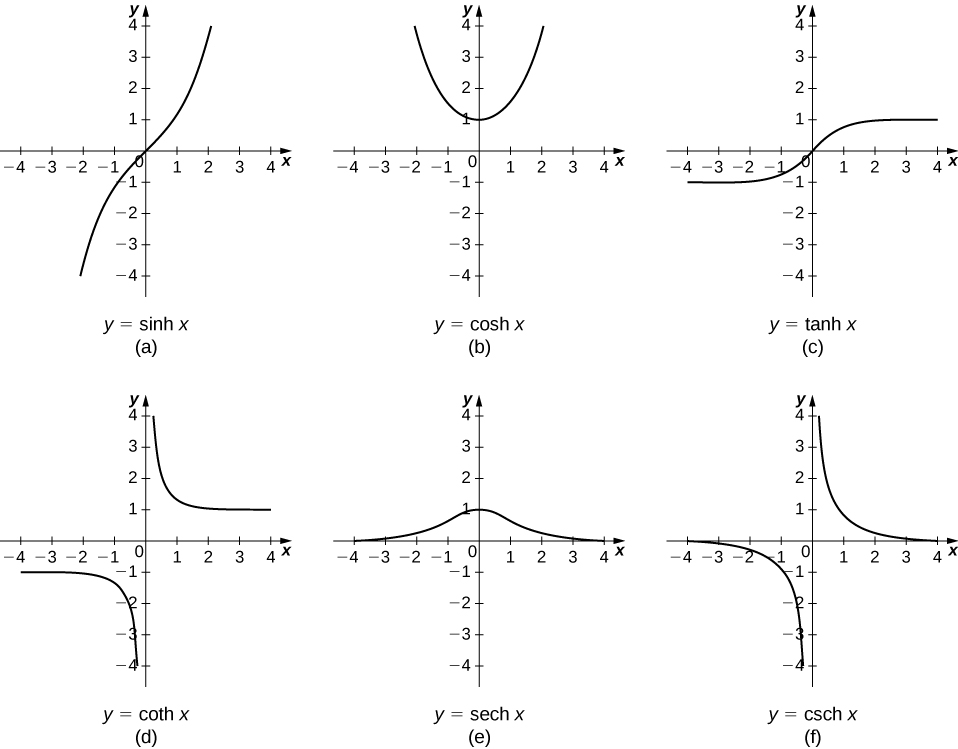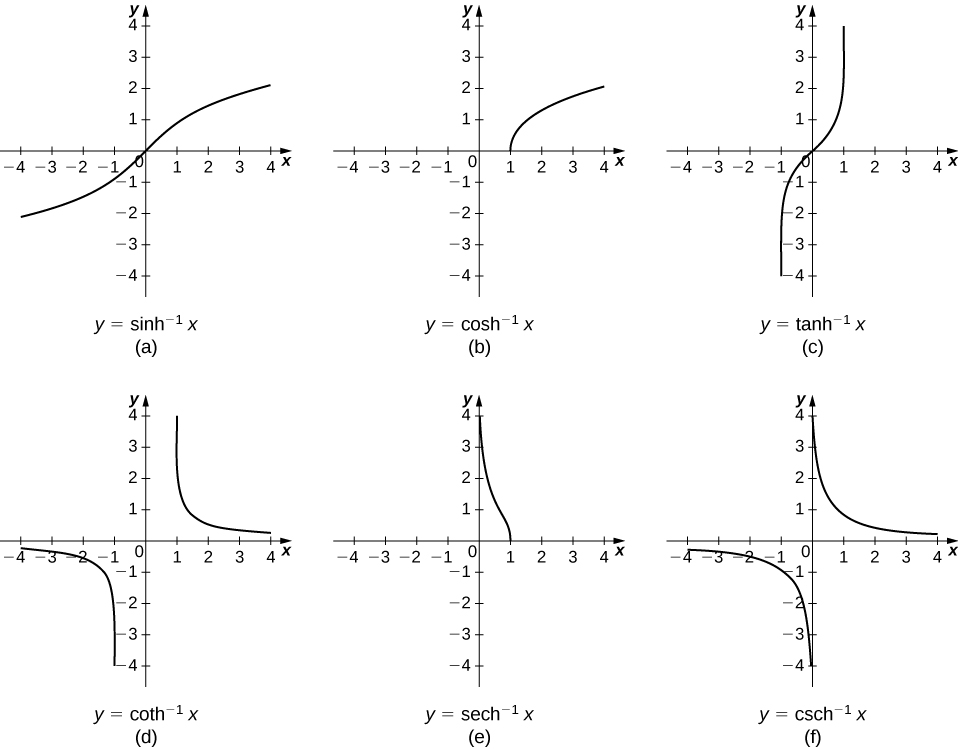## Calculus of Hyperbolic and Inverse Hyperbolic Functions

### Learning Outcomes

• Apply the formulas for derivatives and integrals of the hyperbolic functions
• Apply the formulas for the derivatives of the inverse hyperbolic functions and their associated integrals

## Derivatives and Integrals of the Hyperbolic Functions

Recall that the hyperbolic sine and hyperbolic cosine are defined as

$\text{sinh}x=\dfrac{{e}^{x}-{e}^{\text{−}x}}{2}\text{ and }\text{cosh}x=\dfrac{{e}^{x}+{e}^{\text{−}x}}{2}$

The other hyperbolic functions are then defined in terms of $\text{sinh}x$ and $\text{cosh}x.$ The graphs of the hyperbolic functions are shown in the following figure.Figure 1. Graphs of the hyperbolic functions.

It is easy to develop differentiation formulas for the hyperbolic functions. For example, looking at $\text{sinh}x$ we have

$\begin{array}{cc}\hfill \frac{d}{dx}(\text{sinh}x)& =\frac{d}{dx}(\frac{{e}^{x}-{e}^{\text{−}x}}{2})\hfill \\ & =\frac{1}{2}\left[\frac{d}{dx}({e}^{x})-\frac{d}{dx}({e}^{\text{−}x})\right]\hfill \\ & =\frac{1}{2}\left[{e}^{x}+{e}^{\text{−}x}\right]=\text{cosh}x.\hfill \end{array}$

Similarly, $(\frac{d}{dx})\text{cosh}x=\text{sinh}x.$ We summarize the differentiation formulas for the hyperbolic functions in the following table.

Derivatives of the Hyperbolic Functions
$f(x)$ $\frac{d}{dx}f(x)$
$\text{sinh}x$ $\text{cosh}x$
$\text{cosh}x$ $\text{sinh}x$
$\text{tanh}x$ ${\text{sech}}^{2}x$
$\text{coth}x$ $\text{−}{\text{csch}}^{2}x$
$\text{sech}x$ $\text{−}\text{sech}x\text{tanh}x$
$\text{csch}x$ $\text{−}\text{csch}x\text{coth}x$

Let’s take a moment to compare the derivatives of the hyperbolic functions with the derivatives of the standard trigonometric functions. There are a lot of similarities, but differences as well. For example, the derivatives of the sine functions match: $(\frac{d}{dx}) \sin x= \cos x$ and $(\frac{d}{dx})\text{sinh}x=\text{cosh}x.$ The derivatives of the cosine functions, however, differ in sign: $(\frac{d}{dx}) \cos x=\text{−} \sin x,$ but $(\frac{d}{dx})\text{cosh}x=\text{sinh}x.$ As we continue our examination of the hyperbolic functions, we must be mindful of their similarities and differences to the standard trigonometric functions.

These differentiation formulas for the hyperbolic functions lead directly to the following integral formulas.

$\begin{array}{cccccccc}\hfill \displaystyle\int \text{sinh}udu& =\hfill & \text{cosh}u+C\hfill & & & \hfill \displaystyle\int {\text{csch}}^{2}udu& =\hfill & \text{−}\text{coth}u+C\hfill \\ \hfill \displaystyle\int \text{cosh}udu& =\hfill & \text{sinh}u+C\hfill & & & \hfill \displaystyle\int \text{sech}u\text{tanh}udu& =\hfill & \text{−}\text{sech}u+C\hfill \\ \hfill \displaystyle\int {\text{sech}}^{2}udu& =\hfill & \text{tanh}u+C\hfill & & & \hfill \displaystyle\int \text{csch}u\text{coth}udu& =\hfill & \text{−}\text{csch}u+C\hfill \end{array}$

### Example: Differentiating Hyperbolic Functions

Evaluate the following derivatives:

1. $\frac{d}{dx}(\text{sinh}({x}^{2}))$
2. $\frac{d}{dx}{(\text{cosh}x)}^{2}$

### Try It

Evaluate the following derivatives:

1. $\frac{d}{dx}(\text{tanh}({x}^{2}+3x))$
2. $\frac{d}{dx}(\dfrac{1}{{(\text{sinh}x)}^{2}})$

Watch the following video to see the worked solution to Example: Differentiating Hyperbolic Functions and the above Try It.

### example: Integrals Involving Hyperbolic Functions

Evaluate the following integrals:

1. $\displaystyle\int x\text{cosh}({x}^{2})dx$
2. $\displaystyle\int \text{tanh}xdx$

### Try It

Evaluate the following integrals:

1. $\displaystyle\int {\text{sinh}}^{3}x\text{cosh}xdx$
2. $\displaystyle\int {\text{sech}}^{2}(3x)dx$

Watch the following video to see the worked solution to the above Try It.

## Calculus of Inverse Hyperbolic Functions

Looking at the graphs of the hyperbolic functions, we see that with appropriate range restrictions, they all have inverses. Most of the necessary range restrictions can be discerned by close examination of the graphs. The domains and ranges of the inverse hyperbolic functions are summarized in the following table.

Domains and Ranges of the Inverse Hyperbolic Functions
Function Domain Range
${\text{sinh}}^{-1}x$ $(\text{−}\infty ,\infty )$ $(\text{−}\infty ,\infty )$
${\text{cosh}}^{-1}x$ $(1,\infty )$ $[0,\infty )$
${\text{tanh}}^{-1}x$ $(-1,1)$ $(\text{−}\infty ,\infty )$
${\text{coth}}^{-1}x$ $(\text{−}\infty ,-1)\cup (1,\infty )$ $(\text{−}\infty ,0)\cup (0,\infty )$
${\text{sech}}^{-1}x$ $(0\text{, 1})$ $[0,\infty )$
${\text{csch}}^{-1}x$ $(\text{−}\infty ,0)\cup (0,\infty )$ $(\text{−}\infty ,0)\cup (0,\infty )$

The graphs of the inverse hyperbolic functions are shown in the following figure.Figure 2. Graphs of the inverse hyperbolic functions.

To find the derivatives of the inverse functions, we use implicit differentiation. We have

$\begin{array}{ccc}\hfill y& =\hfill & {\text{sinh}}^{-1}x\hfill \\ \hfill \text{sinh}y& =\hfill & x\hfill \\ \hfill \frac{d}{dx}\text{sinh}y& =\hfill & \frac{d}{dx}x\hfill \\ \hfill \text{cosh}y\frac{dy}{dx}& =\hfill & 1.\hfill \end{array}$

Recall that ${\text{cosh}}^{2}y-{\text{sinh}}^{2}y=1,$ so $\text{cosh}y=\sqrt{1+{\text{sinh}}^{2}y}.$ Then,

$\frac{dy}{dx}=\frac{1}{\text{cosh}y}=\frac{1}{\sqrt{1+{\text{sinh}}^{2}y}}=\frac{1}{\sqrt{1+{x}^{2}}}.$

We can derive differentiation formulas for the other inverse hyperbolic functions in a similar fashion. These differentiation formulas are summarized in the following table.

Derivatives of the Inverse Hyperbolic Functions
$f(x)$ $\frac{d}{dx}f(x)$
${\text{sinh}}^{-1}x$ $\frac{1}{\sqrt{1+{x}^{2}}}$
${\text{cosh}}^{-1}x$ $\frac{1}{\sqrt{{x}^{2}-1}}$
${\text{tanh}}^{-1}x$ $\frac{1}{1-{x}^{2}}$
${\text{coth}}^{-1}x$ $\frac{1}{1-{x}^{2}}$
${\text{sech}}^{-1}x$ $\frac{-1}{x\sqrt{1-{x}^{2}}}$
${\text{csch}}^{-1}x$ $\frac{-1}{|x|\sqrt{1+{x}^{2}}}$

Note that the derivatives of ${\text{tanh}}^{-1}x$ and ${\text{coth}}^{-1}x$ are the same. Thus, when we integrate $1\text{/}(1-{x}^{2}),$ we need to select the proper antiderivative based on the domain of the functions and the values of $x.$ Integration formulas involving the inverse hyperbolic functions are summarized as follows.

$\begin{array}{cccccccc}\hfill \displaystyle\int \frac{1}{\sqrt{1+{u}^{2}}}du& =\hfill & {\text{sinh}}^{-1}u+C\hfill & & & \hfill \displaystyle\int \frac{1}{u\sqrt{1-{u}^{2}}}du& =\hfill & \text{−}{\text{sech}}^{-1}|u|+C\hfill \\ \hfill \displaystyle\int \frac{1}{\sqrt{{u}^{2}-1}}du& =\hfill & {\text{cosh}}^{-1}u+C\hfill & & & \hfill \displaystyle\int \frac{1}{u\sqrt{1+{u}^{2}}}du& =\hfill & \text{−}{\text{csch}}^{-1}|u|+C\hfill \\ \hfill \displaystyle\int \frac{1}{1-{u}^{2}}du& =\hfill & \bigg\{\begin{array}{c}{\text{tanh}}^{-1}u+C\text{ if }|u|<1\hfill \\ {\text{coth}}^{-1}u+C\text{ if }|u|>1\hfill \end{array}\hfill & & & & & \end{array}$

### Example: Differentiating Inverse Hyperbolic Functions

Evaluate the following derivatives:

1. $\frac{d}{dx}({\text{sinh}}^{-1}(\frac{x}{3}))$
2. $\frac{d}{dx}{({\text{tanh}}^{-1}x)}^{2}$

### Try It

Evaluate the following derivatives:

1. $\frac{d}{dx}({\text{cosh}}^{-1}(3x))$
2. $\frac{d}{dx}{({\text{coth}}^{-1}x)}^{3}$

Watch the following video to see the worked solution to the above Try It.

### Example: Integrals Involving Inverse Hyperbolic Functions

Evaluate the following integrals:

1. $\displaystyle\int \frac{1}{\sqrt{4{x}^{2}-1}}dx$
2. $\displaystyle\int \frac{1}{2x\sqrt{1-9{x}^{2}}}dx$

### Try It

Evaluate the following integrals:

1. $\displaystyle\int \frac{1}{\sqrt{{x}^{2}-4}}dx,\text{}x>2$
2. $\displaystyle\int \frac{1}{\sqrt{1-{e}^{2x}}}dx$

Watch the following video to see the worked solution to the above Try It.